# Pontryagin number

(diff) ← Older revision | Latest revision (diff) | Newer revision → (diff)

A characteristic number defined for closed oriented manifolds and assuming rational values. Let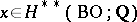be an arbitrary (not necessarily homogeneous) stable characteristic class. For a closed oriented manifoldthe rational numberis called the Pontryagin number ofcorresponding to; hereis the tangent bundle andis the fundamental class of. The Pontryagin number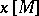depends only on the homogeneous component of degree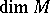of the class. Let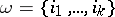be a partition of, i.e. a set of non-negative integers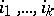such that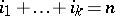and let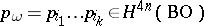. The rational numbersare defined for a closed manifoldof dimensionand all partitionsof the number.

The Pontryagin numbers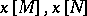of two bordant (in the oriented sense, cf. Bordism) manifolds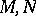are equal: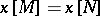(Pontryagin's theorem).

According to this theorem each characteristic classinduces a homomorphism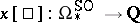, and each element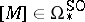induces a homomorphism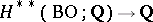,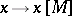. In other words, there is a mappingIf all Pontryagin numbers and Stiefel numbers (cf. Stiefel number) of two oriented closed manifolds coincide, then these manifolds are bordant (in the oriented sense).

A problem similar to the Milnor–Hirzebruch problem for quasi-complex manifolds consists in describing the image of the mapping. The solution of this problem is based on the consideration of Pontryagin numbers in-theory corresponding to the Pontryagin classes (cf. Pontryagin class)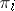in-theory. Let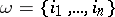be a set of non-negative integers, let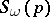andbe the characteristic classes defined by the symmetric series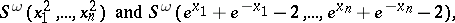respectively; here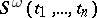is the minimal symmetric polynomial containing the monomials,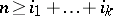. Let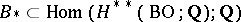be a set of homomorphismsfor which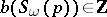,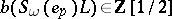for all tuples. Then the image of the homomorphism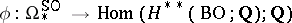coincides with(the Stong–Hattori theorem).

The characteristic numbers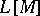andcorresponding to the classes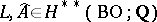are called the-genus and the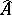-genus of, respectively.

For a closed manifoldof dimension divisible bythe equality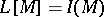holds, where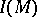is the signature of the manifold, i.e. the signature of the quadratic intersection form defined on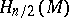,(Hirzebruch's theorem). For a closed spin manifoldof even dimension the spinor index of, i.e. the index of the Dirac operator on, coincides with.

For references see Pontryagin class.

How to Cite This Entry:
Pontryagin number. Encyclopedia of Mathematics. URL: http://encyclopediaofmath.org/index.php?title=Pontryagin_number&oldid=13650
This article was adapted from an original article by A.F. Kharshiladze (originator), which appeared in Encyclopedia of Mathematics - ISBN 1402006098. See original article